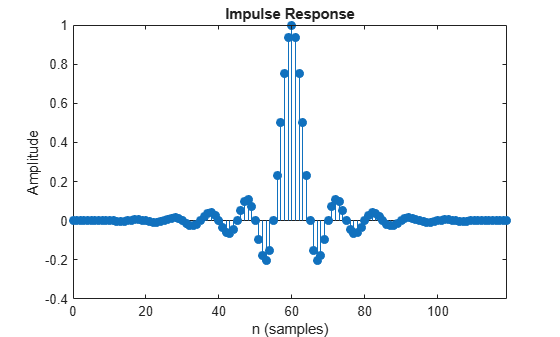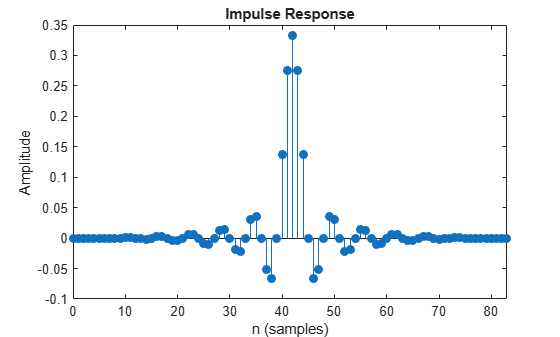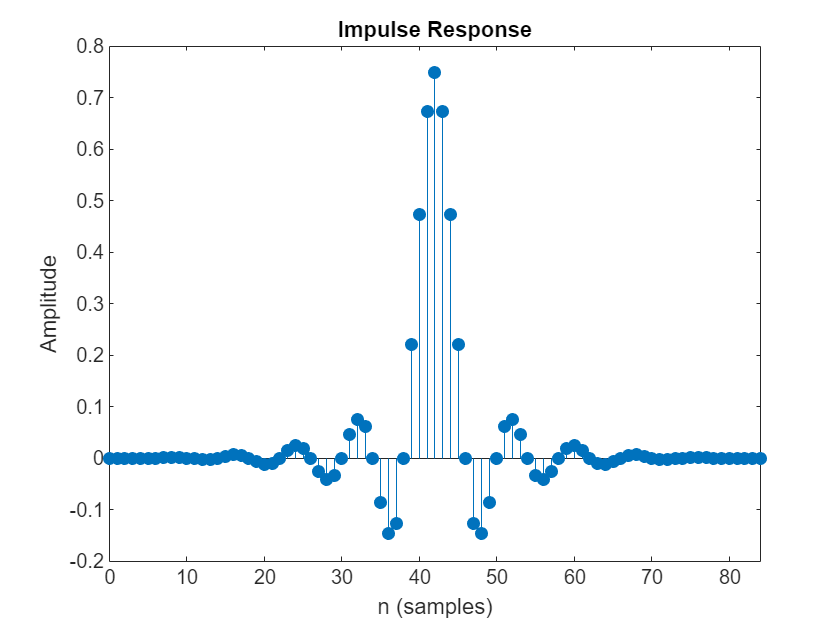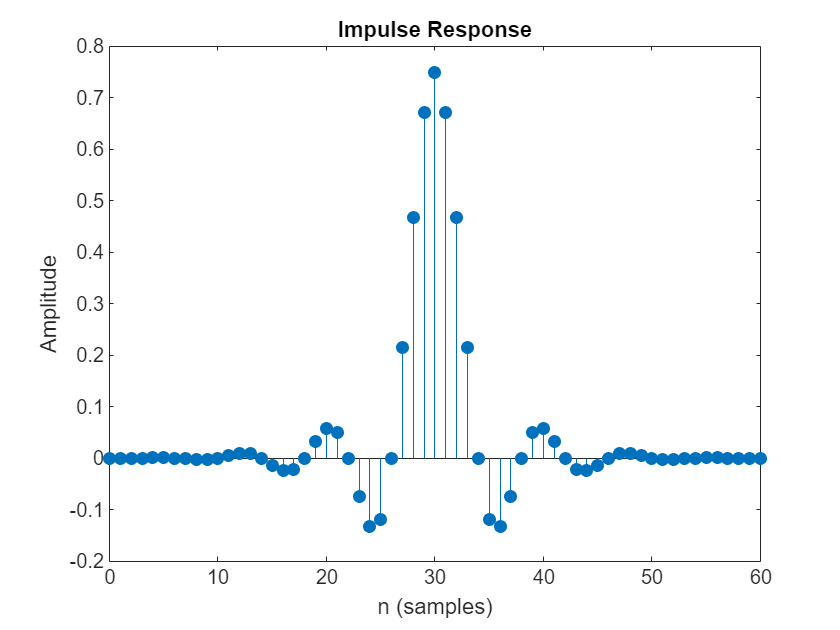# designMultirateFIR

Multirate FIR filter design

## Syntax

``B = designMultirateFIR(L,M)``
``B = designMultirateFIR(L,M,P)``
``B = designMultirateFIR(L,M,TW)``
``B = designMultirateFIR(L,M,P,Astop)``
``B = designMultirateFIR(L,M,TW,Astop)``
``B = designMultirateFIR(___,'SystemObject',flag)``

## Description

example

````B = designMultirateFIR(L,M)` designs a multirate FIR filter with interpolation factor `L` and decimation factor `M`. The output `B` is the vector of designed FIR coefficients. To design a pure interpolator, set M to 1. To design a pure decimator, set L to 1.```

example

````B = designMultirateFIR(L,M,P)` designs a multirate FIR filter with half-polyphase length `P`. By default, the half-polyphase length is `12`.```
````B = designMultirateFIR(L,M,TW)` designs a multirate FIR filter with interpolation factor `L`, decimation factor `M`, and normalized transition width `TW`.```

example

````B = designMultirateFIR(L,M,P,Astop)` designs a multirate FIR filter with stopband attenuation `Astop`. By default, the stopband attenuation is 80 dB.```
````B = designMultirateFIR(L,M,TW,Astop)` designs a multirate FIR filter with interpolation factor `L`, decimation factor `M`, normalized transition width `TW`, and stopband attenuation `Astop`, specified in dB.```
````B = designMultirateFIR(___,'SystemObject',flag)` returns a vector of filter coefficients `B` if the flag is set to `false`, or a multirate filter System object™ if the `flag` is set to `true`.```

## Examples

collapse all

To design an FIR Interpolator using the `designMultirateFIR` function, you must specify the interpolation factor of interest (usually greater than 1) and a decimation factor equal to 1. You can use the default half-polyphase length of 12 and the default stopband attenuation of 80 dB. Alternately, you can also specify the half-polyphase length and stopband attenuation values.

Design an FIR interpolator with interpolation factor set to 5. Use the default half-polyphase length of 12 and the default stopband attenuation of 80 dB.

```b = designMultirateFIR(5,1); fvtool(b,'impulse')```To design an FIR Decimator using the `designMultirateFIR` function, you must specify the decimation factor of interest (usually greater than 1) and an interpolation factor equal to 1. You can use the default half-polyphase length of 12 and the default stopband attenuation of 80 dB. Alternately, you can also specify the half-polyphase length and stopband attenuation values. Design an FIR decimator with decimation factor set to 3, and half-polyphase length set to 14. Use the default stopband attenuation of 80 dB.

```b = designMultirateFIR(1,3,14); fvtool(b,'impulse');```To design an FIR Rate Converter using the `designMultirateFIR` function, you must specify the interpolation and decimation factors of interest (usually greater than 1). In addition, you can specify either the half-polyphase length and stopband attenuation values, or the normalized transition width and stopband attenuation values.

Design an FIR rate converter with interpolation factor set to 3, decimation factor set to 4, half-polyphase length set to 14, and stopband attenuation set to 90 dB.

```b = designMultirateFIR(3,4,14,90); fvtool(b,'impulse');```Design an FIR rate converter with interpolation factor set to 3, decimation factor set to 4, normalized transition width set to 0.2, and stopband attenuation set to 90 dB.

```bTW = designMultirateFIR(3,4,0.2,90); fvtool(bTW,'impulse');```Set the `'SystemObject'` flag to `true` in the `designMultirateFIR` function to design a multirate filter object. The design parameters specified in the function determine the type of System object the function designs.

In this example, the function designs a polyphase FIR interpolator System object™. For more details, see `dsp.FIRInterpolator`.

Create a `dsp.FIRInterpolator` object with the interpolation factor equal to 5, transition width equal to 0.01, and stopband attenuation equal to 60 dB. Set the `'SystemObject' `flag to `true `to design a multirate filter object.

```firInterp = designMultirateFIR(5,1,0.01,60,'SystemObject',true); fvtool(firInterp)```Compute the cost of implementing the filter.

`cost(firInterp)`
```ans = struct with fields: NumCoefficients: 582 NumStates: 145 MultiplicationsPerInputSample: 582 AdditionsPerInputSample: 578 ```

Measure the frequency response characteristics of the filter object.

`measure(firInterp)`
```ans = Sample Rate : N/A (normalized frequency) Passband Edge : 0.195 3-dB Point : 0.19884 6-dB Point : 0.2 Stopband Edge : 0.205 Passband Ripple : 0.016474 dB Stopband Atten. : 60.1827 dB Transition Width : 0.01 ```

## Input Arguments

collapse all

Interpolation factor, specified as a positive scalar integer. To design a decimator only, set L to `1`.

Example: `2`

Data Types: `single` | `double` | `int8` | `int16` | `int32` | `int64` | `uint8` | `uint16` | `uint32` | `uint64`

Decimation factor, specified as a positive scalar integer. To design an interpolator only, set M to `1`.

Example: `2`

Data Types: `single` | `double` | `int8` | `int16` | `int32` | `int64` | `uint8` | `uint16` | `uint32` | `uint64`

Half-polyphase length, specified as a positive scalar integer.

Example: `12`

Example: `20`

Data Types: `single` | `double` | `int8` | `int16` | `int32` | `int64` | `uint8` | `uint16` | `uint32` | `uint64`

Normalized transition width of the multirate FIR filter, specified as a real scalar in the range (0 1).

Data Types: `single` | `double` | `int8` | `int16` | `int32` | `int64` | `uint8` | `uint16` | `uint32` | `uint64`

Stopband attenuation in dB, specified as a nonnegative real scalar greater than or equal to `0`.

Example: `0.0`

Example: `80.5`

Data Types: `single` | `double` | `int8` | `int16` | `int32` | `int64` | `uint8` | `uint16` | `uint32` | `uint64`

System object flag set to:

Data Types: `logical`

## Output Arguments

collapse all

Multirate FIR filter coefficients, returned as a real-valued N-length vector.

If both L and M are equal to 1, then N equals 1.

If `max(L,M)` > 1, then N = 2*P*R, where P is the half-polyphase length and R is defined by the following equations:

• If L > 1, R = L.

• If L = 1, R = M.

For more details, see the Algorithms section.

When the `'SystemObject'` flag is set to `true`, the function returns one of the following multirate filter System objects:

Data Types: `double`

## Algorithms

`designMultirateFIR` designs an (N – 1)th order, Rth band Nyquist FIR filter using the N-length Kaiser window vector to window the truncated impulse response of the FIR filter.

Filter length, N is defined as N = 2*P*R and R is defined as explained in B.

The truncated impulse response d(n) is delayed by N/2 samples to make it causal. The truncated and delayed impulse response is given by:

`$d\left(n-N/2\right)=\frac{\mathrm{sin}\left({w}_{c}\left(n-N/2\right)\right)}{\pi \left(n-N/2\right)},\text{ }n=0,\cdots ,\frac{N}{2},\cdots ,N$`

where ${w}_{c}=\pi /R$.

For every Rth band, the impulse response of the Nyquist filters is exactly zero. Because of this property, when Nyquist filters are used for pure interpolation, the input samples remain unaltered after interpolating.

A Kaiser window is used because of its near-optimum performance while providing a robust way of designing a Nyquist filter. The window depends on two parameters: length N + 1 and shape parameter β.

The Kaiser window is defined by:

`$w\left(n\right)=\frac{{I}_{0}\left(\beta \sqrt{1-{\left(\frac{n-N/2}{N/2}\right)}^{2}}\right)}{{I}_{0}\left(\beta \right)},\text{ }\text{ }0\le n\le N,$`

where I0 is the zeroth-order modified Bessel function of the first kind.

The shape parameter β is calculated from:

where Astop is the stopband attenuation in dB.

The windowed impulse response is given by

`$h\left(n\right)=w\left(n\right)d\left(n-N/2\right)=w\left(n\right)\frac{\mathrm{sin}\left({w}_{c}\left(n-N/2\right)\right)}{\pi \left(n-N/2\right)},\text{ }n=0,\cdots ,\frac{N}{2},\cdots ,N$`

h(n) for n = 0,..,N/2,...N are the coefficients of the multirate filter. These coefficients are defined by the interpolation factor, L, and decimation factor, M.

 Orfanidis, Sophocles J. Introduction to Signal Processing. Upper Saddle River, NJ: Prentice-Hall, 1996.

## SupportGet trial now